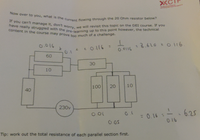# Ohms Law problem

#### Dancoates

##### New member
Hello, sorry if this is the wrong place I cannot find Physics.

I have a problem here and I cannot figure out how to get to the given answer. I can work out the parralel but it is asking for the current through the 20 ohm resistor.

See attached.

Thanks
Dan

#### Attachments

• 621 KB Views: 5

#### Subhotosh Khan

##### Super Moderator
Staff member
Hello, sorry if this is the wrong place I cannot find Physics.

I have a problem here and I cannot figure out how to get to the given answer. I can work out the parralel but it is asking for the current through the 20 ohm resistor.

See attached.

Thanks
Dan
Calculate the TOTAL resistance of the circuit first (for which you will need to calculate the equivalent resistances of the parallel parts first).•toponlinetool and topsquark

#### Romsek

##### Full Member
Ok so you say you've worked out the circuit to the point that you've got the current flowing into the parallel network of 3 resistors at the right.

Let's just call this $$\displaystyle i$$.

You know that this parallel network is equivalent to a single resistor of $$\displaystyle \dfrac{25}{4}$$ ohms.
There for the voltage across this network to ground is $$\displaystyle V=\dfrac{25i}{4}$$ volts.
This voltage is the same for each resistor in the parallel network.

Therefore the current going through the 20 ohm resistor is $$\displaystyle i_{20} = \dfrac{V}{20} = \dfrac{25i}{80} = \dfrac{5i}{16}$$ amps.

•Subhotosh Khan